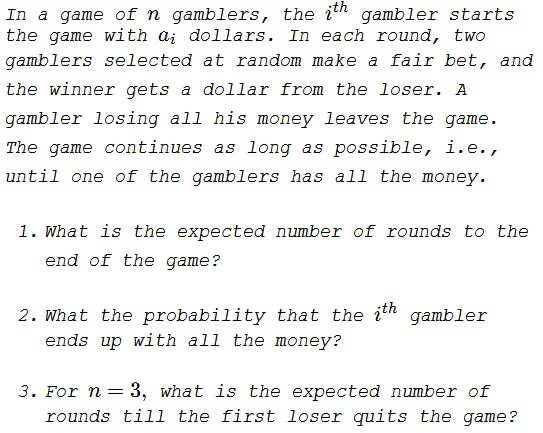# Gambling in a Company

### ProblemThe expected number of rounds is $\displaystyle\sum_{i\lt j}a_ia_j.$

The probability that the $i^{th}$ gambler ends up with all the money $\displaystyle \frac{a_i}{a_1+a_2+\ldots+a_n}.$

For $n=3,$ the expected number of rounds till the first loser quits the game is $\displaystyle \frac{3a_1a_2a_3}{a_1+a_2+a_3}.$

### The $n=2$ Analogue

The problem is an obvious extension of the game of two players. The latter is usually modeled by the well-known one-dimensional walk where a point on an axis moves one step at a time - left or right - with probabilities $\displaystyle p=q=\frac{1}{2}.$ The two players start with the capitals of, say, $u$ and $v$ dollars and lose or win one dollar from the other player with the probability of $\displaystyle\frac{1}{2}.$ On average, the game lasts $uv$ rounds in which the probabilities of winning are $\displaystyle\frac{u}{u+v}$ and $\displaystyle\frac{v}{u+v},$ respectively.

### Definitions

Let $\mathbf{u}=(u_1,u_2,\ldots, u_n)$ denote the state of the game where the $i^{th}$ player owns $u_i\ge 0$ dollars. We'll write $|\mathbf{u}|=u_1+u_2+ \ldots+u_n.$ The quantity $a=|\mathbf{u}|$ remains unchanged during the game.

Let $N(\mathbf{u})$ be the set of all states that can be attained from $\mathbf{u}$ in one round. If $\mathbf{u}$ has $k$ non-zero components then $|N(\mathbf{u})|=k(k-1).$ Define $V(\mathbf{u})$ as the set of all states that could be attained starting with $\mathbf{u}):$

$V(\mathbf{u})=\left\{\mathbf{v}:\, \forall i\,v_i\ge 0, |\mathbf{u}|=|\mathbf{v}|, (u_i=0)\,\Rightarrow \,(v_i=0)\right\}.$

### Solution 1, Part 1

Let $R(\mathbf{u})$ be the expected number of rounds, starting with $\mathbf{u},$ till one of the players has all the money. (We are to prove that $\displaystyle\mathbf{a}=\sum_{j\lt j}a_ia_j.)$ There is a recurrent relation: $R(\mathbf{u})$ is the average of all $\mathbf{x}\in N(\mathbf{u})$ plus $1.$ We also have boundary conditions: $R(\mathbf{u})=0$ only if all but one component of $\mathbf{u}$ are zero.

The function $\displaystyle R(\mathbf{u})=\sum_{i\lt j}a_ia_j$ satisfies the recurrence and the boundary condition. Indeed, for any two components $u$ and $v,$ the product $uv$ appears in every term of the sums over the neighborhood $N(\mathbf{u}),$ the same holds for $-1;$ the linear terms $u$ and $v$ cancel out. We only need to prove that no other function satisfies the recurrence, along with the boundary conditions.

If there are two such solutions then the difference at any point is the average of its values in the point's neighborhood and, therefore, could not be either maximum or minimum (in the neighborhood), unless the function is constant. Then the function is constant over the whole play field $V=V(\mathbf{x})$ and, being $0$ on the boundary is $0$ on $V,$ meaning that the two solutions coincide.

### Solution 1, Part 2

Let $p_i(\mathbf{u})$ be the probability that the $i^{th}$ player ends up with all the money, starting with the state $\mathbf{u}.$ (We have to prove that $\displaystyle p_i(\mathbf{u})=\frac{u_i}{|\mathbf{u}|}.)$ $p_i(\mathbf{u})$ is the average of all $p_i(\mathbf{x}),$ for $x\in N(\mathbf{u}),$ with the following boundary conditions: $p_i(\mathbf{u})=0$ if $u_i=0$ and $p_i(\mathbf{u})=1$ if $u_i=a,$ so that all other components vanish automatically.

As before, all it takes is to prove uniqueness.

### Solution 1, Part 3

Here the recurrence is exactly the same as for the first question, but the boundary condition is different: the game stops when one of the three components vanishes.

### Solution 2, Part 1

Let $\displaystyle a=\sum_{i=1}^na_i$ be the total capital at play. Let $R_i$ be the number of rounds played by the $i^{th}$ gambler. The remaining $(n-1)$ gamblers are undistinguishable from a single gambler with $(a-a_j)$ dollars. Hence we only need to consider two gamblers, for which $E(R_i)=a_i(a-a_i).$ The total number of rounds is $\displaystyle R=\frac{1}{2}\sum_{i=1}^nR_i,$ hence

$\displaystyle E(R)=\frac{1}{2}\sum_{i=1}^na_i(a-a_i)=\sum_{i\lt j}a_ia_j.$

### Solution 2, Part 2

Consider $a$ players with $1$ dollar each. Before the first round, the probability of winning the game is uniform among the players: $\displaystyle\frac{1}{a}.$ Partition the players into $n$ teams, so that the $i^{th}$ team has $a_i$ members/dollars. Then the probability of the $i^{th}$ team (i.e., gambler) winning the game is $\displaystyle p_i=a_i\cdot\frac{1}{a}=\frac{a_i}{a}.$

### Acknowledgment

This problem 53 from B. Bollobás, The Art of Mathematics: Coffee Time in Memphis, Cambridge University Press, 2006, 149-150.

The second set of solutions is by Hélvio Vairinhos.